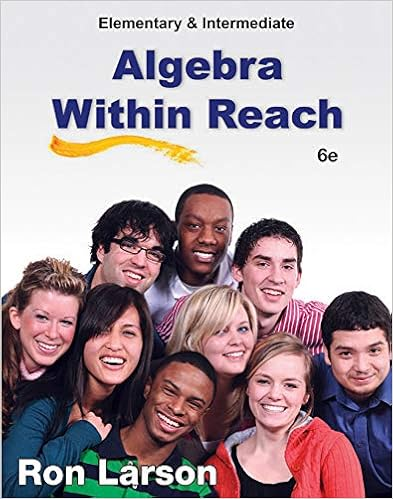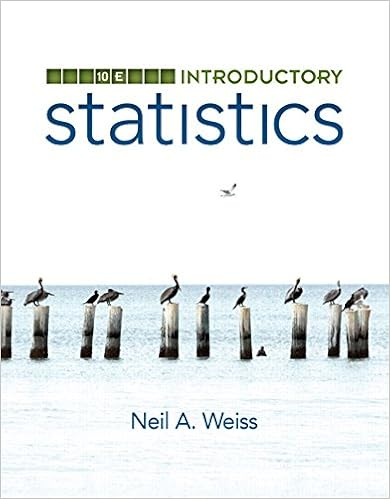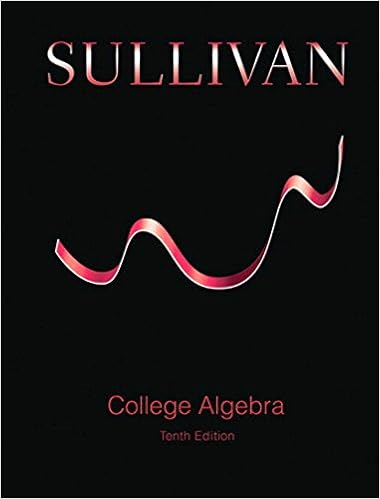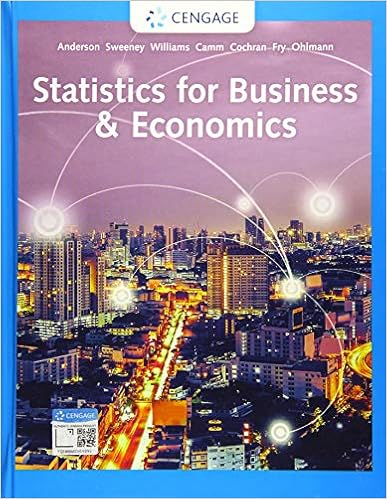# FinalExamQuestions - MAP 103 FINAL EXAM SAMPLE QUESTIONS...

• Notes
• 2
• 100% (1) 1 out of 1 people found this document helpful

This preview shows page 1 - 2 out of 2 pages.

The preview shows page 1 - 2 out of 2 pages.
MAP 103 FINAL EXAM SAMPLE QUESTIONS(1) Expressyas a function ofxfor the expression: log3y= 2 + 4 log3(2x).(2) Supposef(x) =x+ 1, g(x) = 2x-1,andh(2) = 3.Findfgh(2).(3) Letf(x) =12x-13.Write a formula for the inverse and check thatff-1(x) =x.(4) Determine if the following are true or false. CircleTfor true orFfor false. If false, providea counter-example.(a)T|F: log(a+b) = log(a) + log(b) fora, b0(b)T|F: 2x·2x= 22x(c)T|F: logrx2y3z4!=12[log(2x) + log(3y)-log(4z)](d)T|F: log(ab) = log(a) log(b) fora, b0(5) Evaluate: log22 + log22 + log222+ log 212.(6) Letf(x) =x2+ 6x+ 9.(a) What are the coordinates for the vertex?(b) How many values ofxsatisfy the equationf(x) = 0?(c) What is the equation of the line that passes through the vertex that has slope 0?(d) What is the new equation forf(x) after the transformationf(x+ 1) + 1?(7) A catapult launches a rock into the air. The motion of the rock is modeled by the equationm(t) =-1000t2+18000t+26000.Suppose that down range there is a castle with a defensivewall 108,000 feet high.
##### We have textbook solutions for you!The document you are viewing contains questions related to this textbook.
Chapter 3 / Exercise 94
Elementary and Intermediate Algebra: Algebra Within Reach
LarsonExpert VerifiedBrowse all Textbook Solutions

Course Hero member to access this document

End of preview. Want to read all 2 pages?

Course Hero member to access this document

Term
Fall
Professor
Staff
Tags
Rational function
##### We have textbook solutions for you!
The document you are viewing contains questions related to this textbook.The document you are viewing contains questions related to this textbook.
Chapter 3 / Exercise 94
Elementary and Intermediate Algebra: Algebra Within Reach
LarsonExpert Verified
•••